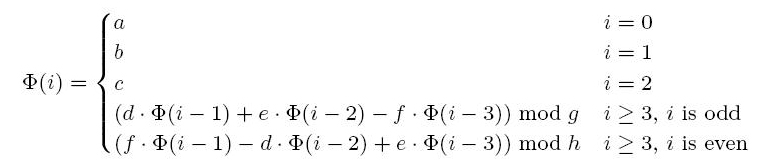Online JudgeProblem SetAuthorsOnline ContestsUser
Web Board
F.A.Qs
Statistical Charts
Problems
Submit Problem
Online Status
Prob.ID:
Register
Authors ranklist
Current Contest
Past Contests
Scheduled Contests
Award Contest
Register
Language:
A Mysterious Function
 Time Limit: 1000MS Memory Limit: 65536K Total Submissions: 4161 Accepted: 2871

Description

For any integers p and q with q > 0, define p mod q to be the integer r with 0 <= r <= q −1 such that p−r is divisible by q. For example, we have
109 mod 10 = 9
−7 mod 3 = 2
−56 mod 7 = 0

Let Φ be a function defined recursively as follows.where a, b, c, d, e, f, g, h are integers with 0 < a, b, c, d, e, f, g, h <= 1000. One can easily see that 0 <= Φ(i) <= 1000 holds for any integer i >= 0. Now for any given integers a, b, c, d, e, f, g, h, i with 0 < a, b, c, d, e, f, g, h, i <= 1000, you are asked to write a program to output

Φ(i). (Hint: a direct recursive implementation of the above recurrence

relation is likely to run forever for large i.)

Input

The first line contains the number n of test cases. Each of the following n lines contains the sequence a, b, c, d, e, f, g, h, i of integers.

Output

For each test case, your program has to output the correct value of Φ(i).

Sample Input

```3
1 2 3 4 5 6 7 8 9
11 12 13 14 15 16 17 18 19
321 322 323 324 325 326 327 328 329```

Sample Output

```4
0
90```

Source

[Submit]   [Go Back]   [Status]   [Discuss]Home PageGo BackTo top